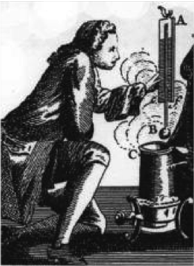Temperature and Heat

Equation

C = (F – 32) * 5/9

3.1

Equation

F = (C * 9/5) + 32

3.2

Equation

C = K - 273

3.3

Equation

K = C + 273

3.4

Equation

F = (K - 273) * 9/5 + 32

3.5

Equation

K = (F - 32) * 5/9 + 273

3.6

Measuring Temperature

There are three common temperature scales, named after their inventors: Fahrenheit, Celsius and Kelvin. Each has a different starting point, and two have different size units or degrees.

Temperature is measured by comparison to a physical process. Two of the three temperature scales use the freezing and boiling points of water. These are great standards because any thermometer manufacturer can freeze and boil water and thus independently calibrate their instruments so they all agree.Fahrenheit and his thermometer, engraving from WikipediaYou might ask why the Fahrenheit scale uses 32° for freezing rather than 0°. Daniel Gabriel Fahrenheit, a Polish glassblower who lived in Holland and invented the mercury thermometer in 1714, is responsible. He made the zero point of his scale be the temperature where a mixture of ice, water and a salt reached equilibrium. With that being set at 0° he found that the freezing of water occurred 32° warmer.  Other experiments showed that water boils at a temperature 180° higher than it freezes, so the boiling point temperature is 32° + 180° = 212°.

Incidentally, Fahrenheit became an orphan at the age of 15 when both his parents died after eating poisonous mushrooms.

Fortunately, other scientists soon selected a more rational temperature scale, setting the freezing of water at 0° and the boiling point at 100°. The Swedish astronomer Anders Celsius invented this Centigrade scale of 100 steps in 1742. For two centuries it was called the Centigrade scale but in 1948 was renamed in his honor as the Celsius scale.  Virtually everyone on Earth uses the Celsius scale, just as nearly everyone uses the metric scale for mass and length. The exceptions, who continue to use °F, are the United States and Belize…

Because the °C scale has 100 divisions or degrees between freezing and boiling, while the °F scale has 180 divisions, the °F degrees are about half (actually 5/9th) the size as the °C ones. This is of no importance except that it, and the different temperatures for the freezing point, makes conversion between the two scales awkward. Students often are forced to convert between the two scales on SAT exams so it is necessary to show how to do it:

Celsius = (Fahrenheit minus 32°) x 5/9
C = (F – 32) * 5/9

An example: What does 20 °F = in °C?      C = (20-32)*5/9 = -12*5/9 = -7 °C

Fahrenheit = (Centigrade x 9/5) + 32° F = (C * 9/5) + 32

An example:  What does 20 °C = in °F?     F = (20*9/5) + 32 = 36 + 32 = 68 °F

Now try this: What does -40 °C = in °F?    ?

(If you live in North Dakota or on Mars you have probably already experienced the answer.)

Lord K, from WikipediaThe fraction 5/9 is the ratio of the size of the F to the C degree, and 9/5 is vice versa. The + or – 32 is the correction to the freezing of water temperature. If the USA would accept the Celsius scale students would not have to worry about such conversions.

Nearly one hundred years after Celsius a growing understanding of physics led Lord Kelvin in England to propose a temperature scale based on the point at which atoms and molecules stop moving. This absolute zero, where no further heat can be removed, was set as the zero point for what became known as the Kelvin temperature scale. It uses degrees of the same size as the Celsius scale, so that the freezing point of water was 273 K higher. Notice I didn’t write 273°K higher - the degree symbol (°) is not used for measurements of temperature in the Kelvin scale. All temperatures are positive on the Kelvin scale. Scientists never use the F scale, preferring the metric simplicity of K or C.

Converting to and from the K scale is easy for C temperatures:

Centigrade = Kelvin -273°Kelvin = Centrigrade + 273°  C = K - 273K = C + 273

The F conversion is messier:

Fahrenheit = (Kelvin-273) x 9/5 + 32°Kelvin = (Fahrenheit -32°) x 5/9 +273°
F = (K - 273) * 9/5 + 32
K = (F - 32) * 5/9 + 273

Now try this: The exhaust from the main engines of the Space Shuttle is hot – the measured temperature is 3,200 °C; what does that equal in °F and K?